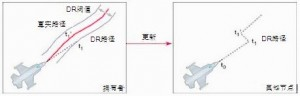# 网络游戏的移动同步（二）状态更新及航位预测法

## 状态更新

 `````` 1 2 3 4 5 6 7 8 9 10 11 12 13 14 15 16 17 18 19 20 `````` ``````// 获取输入 // 在通过键盘获取输入更新最新速度前 var lastVelocity:Vector2 = localPlayer.velocity.clone(); var lastAcceleration:Vector2 = localPlayer.acceleration.clone(); if (lastVelocity.x != localPlayer.velocity.x || lastVelocity.y != localPlayer.velocity.y || lastAcceleration.x != localPlayer.acceleration.x || lastAcceleration.y != localPlayer.acceleration.y) { update = true; } if (update) { var p:PlayerStatePack = new PlayerStatePack(); p.velocity = localPlayer.velocity.clone(); p.position = localPlayer.position.clone(); p.acceleration = localPlayer.acceleration.clone(); p.time = getTimer(); client.send(p); } ``````

Network1.swf`````` 1 2 3 4 5 6 7 8 9 10 11 12 `````` ``````// dr位置 var drPosition:Vector2 = new Vector2(); if (lastState) { var e:Number = (getTimer() - lastStateTime) / 1000; // s = v0t + 0.5 * a * t^2; drPosition.addVectors(lastState.position, lastState.velocity.clone().multiply(e).add(lastState.acceleration.clone().divide(2).multiply(e * e))); } // 阈值 var threshold:Number = 5 * 5; if (localPlayer.position.distSQ(drPosition) > threshold) { update = true; } ``````

Network2.swf